# A simple and precise method for pricing convertible bond with credit risk

## Abstract

This article presents a new framework for valuing hybrid defaultable financial instruments, for example, convertible bonds. In contrast to previous studies, the model relies on the probability distribution of a default jump rather than the default jump itself, as the default jump is usually inaccessible. As such, the model can back out the market prices of convertible bonds. A prevailing belief in the market is that convertible arbitrage is mainly due to convertible underpricing. Empirically, however, we do not find evidence supporting the underpricing hypothesis. Instead, we find that convertibles have relatively large positive gammas. As a typical convertible arbitrage strategy employs delta-neutral hedging, a large positive gamma can make the portfolio highly profitable, especially for a large movement in the underlying stock price.

## INTRODUCTION

A company can raise capital in financial markets either by issuing equities, bonds or hybrids (such as convertible bonds). From an investor’s perspective, convertible bonds with embedded optionality offer certain benefits of both equities and bonds – like the former, they have the potential for capital appreciation and like the latter, they offer interest income and safety of principal. The convertible bond market is of primary global importance.

There is a rich literature on the subject of convertible bonds. Arguably, the first widely adopted model among practitioners is the one presented by Goldman Sachs (1994) and then formalized by Tsiveriotis and Fernandes (1998). The Goldman Sachs’ solution is a simple one-factor model with an equity binomial tree to value convertible bonds. The model considers the probability of conversion at every node. If the convertible is certain to remain a bond, it is then discounted by a risky discount rate that reflects the credit risk of the issuer. If the convertible is certain to be converted, it is then discounted by the risk-free interest rate that is equivalent to default-free.

Tsiveriotis and Fernandes (1998) argue that in practice, one is usually uncertain as to whether the bond will be converted, and thus propose dividing convertible bonds into two components: a bond part that is subject to credit risk and an equity part that is free of credit risk. A simple description of this model and an easy numerical example in the context of a binomial tree can be found in Hull (2003).

Grimwood and Hodges (2002) indicate that the Goldman Sachs model is incoherent because it assumes that bonds are susceptible to credit risk but equities are not. Ayache et al (2003) conclude that the Tsiveriotis–Fernandes model is inherently unsatisfactory because of its unrealistic assumption of stock prices being unaffected by bankruptcy. To correct this weakness, Davis and Lischka (1999), Andersen and Buffum (2004), Bloomberg (2009) and Carr and Linetsky (2006), and so on propose a jump-diffusion model to explore defaultable stock price dynamics. They all believe that under a risk-neutral measure, the expected rate of return on a defaultable stock must be equal to the risk-free interest rate. The jump-diffusion model characterizes the default time/jump directly.

The jump-diffusion model was first introduced by Merton (1976) in the market risk context for modeling asset price behavior that incorporates small day-to-day diffusive movements together with larger randomly occurring jumps. Over the last decade, people attempt to propagate the model from the market risk domain to the credit risk arena. At the heart of the jump-diffusion models lies the assumption that the total expected rate of return to the stockholders is equal to the risk-free interest rate under a risk-neutral measure.

Although we agree that under a risk-neutral measure the market price of risk and risk preferences are irrelevant to asset pricing (Hull, 2003) and thereby the expectation of a risk-freeFootnote 1 asset grows at the risk-free interest rate, we are not convinced that the expected rate of return on a defaultable asset must be also equal to the risk-free rate. We argue that unlike market risk, credit risk actually has a significant impact on asset prices. This is why regulators, such as International Accounting Standards Board (IASB), Basel Committee on Banking Supervision (BCBS) and so on require financial institutions to report a credit value adjustment (CVA) in addition to the risk-free mark-to-market (MTM) value to reflect credit risk (Xiao, 2013). By definition, a CVA is the difference between the risk-free value and the risky value of an asset/portfolio subject to credit risk. CVA implies that the risk-free value should not be equal to the risky value in the presence of default risk. As a matter of fact, we will prove that the expected return of a defaultable asset under a risk-neutral measure actually grows at a risky rate rather than the risk-free rate. This conclusion is very important for risky valuation.

Because of their hybrid nature, convertible bonds attract different type of investors. Especially, convertible arbitrage hedge funds play a dominant role in primary issues of convertible debt. In fact, it is believed that hedge funds purchase 70 to 80 per cent of the convertible debt offered in primary markets. A prevailing belief in the market is that convertible arbitrage is mainly due to convertible underpricing (that is, the model prices are on average higher than the observed trading prices) (see Ammann et al, 2003; Choi et al, 2009; Loncarski et al, 2009; Calamos, 2011; and so on). However, Agarwal et al (2007) and Batta et al (2010) argue that the excess returns from convertible arbitrage strategies are not mainly due to underpricing, but rather partly due to illiquidity. Calamos (2011) believes that arbitrageurs in general take advantage of volatility. A higher volatility in the underlying equity translates into a higher value of the equity option and a lower conversion premium. Multiple views reveal the complexity of convertible arbitrage, involving taking positions in the convertible bond and the underlying asset that hedges certain risks but leaves managers exposed to other risks for which they reap a reward.

This article makes a theoretical and empirical contribution to the study of convertible bonds. In contrast to the above mentioned literature, we present a model that is based on the probability distribution (or intensity) of a default jump (or a default time) rather than the default jump itself, as the default jump is usually inaccessible (see Duffie and Huang, 1996; Jarrow and Protter, 2004; and so on).

We model both equities and bonds as defaultable in a consistent way. When a firm goes bankrupt, the investors who take the least risk are paid first. Secured creditors have the best chances of seeing the value of their initial investments come back to them. Bondholders have a greater potential for recovering some their losses than stockholders who are last in line to be repaid and usually receive little, if anything. The default proceedings provide a justification for our modeling assumptions: Different classes of securities issued by the same company have the same default probability but different recovery rates. Given this model, we are able to back out the market prices.

Valuation under our risky model can be solved by common numerical methods, such as, Monte Carlo simulation, tree/lattice approaches or partial differential equation (PDE) solutions. The PDE algorithm is elaborated in this article, but of course the methodology can be easily extended to tree/lattice or Monte Carlo.

Using the model proposed, we conduct an empirical study of convertible bonds. We obtain a data set from an investment bank. The data set contains 164 convertible bonds and 2 years of daily market prices as well as associated interest rate curves, credit curves, stock prices, implied Black–Scholes volatilities and recovery rates.

The most important parameter to be determined is the volatility input for valuation. A common approach in the market is to use the at-the-money (ATM) implied Black–Scholes volatility to price convertible bonds. However, most liquid stock options have relatively short maturates (rarely more than 8 years). As a result, some authors, such as Ammann et al (2003), Loncarski et al (2009), Zabolotnyuk et al (2010), have to make do with historical volatilities. Therefore, we segment the sample into two sets according to maturity: a short-maturity class (0~8 years) and a long-maturity class (>8 years). For the short-maturity class, we use the ATM implied Black–Scholes volatility for valuation, whereas for the long-maturity class, we calculate the historical volatility as the annualized standard deviation of the daily log returns over the last 2 years and then price the convertible bond based on this real-world volatility.

The empirical results show that the model prices fluctuate randomly around the market prices, indicating the model is quite accurate. Our empirical evidence does not support a systematic underpricing hypothesis. A similar conclusion is reached by Ammann et al (2008) who use a Monte Carlo simulation approach. Moreover, market participants almost always calibrate their models to the observed market prices using implied convertible volatilities. Therefore, underpricing may not be the main driver of profitability in convertible arbitrage.

It is useful to examine the basics of the convertible arbitrage strategy. A typical convertible bond arbitrage employs delta-neutral hedging, in which an arbitrageur buys a convertible bond and sells the underlying equity at the current delta (see Choi et al, 2009; Loncarski et al, 2009; and so on). With delta-neutral positions, the sign of gamma is important. If gamma is negative, the portfolio profits so long as the underlying equity remains stable. If gamma is positive, the portfolio will profit from large movements in the stock price in either direction (Somanath, 2011).

We study the sensitivities of convertible bonds and find that convertible bonds have relatively large positive gammas, implying that convertible arbitrage can make a big profit on a large upside or downside movement in the underlying stock price. Since convertible bonds are issued mainly by start-up or small companies (while more established firms rely on other means of financing), the chance of a large movement in either direction is very likely. Even for very small movements in the underlying stock price, profits can still be generated from the yield of the convertible bond and the interest rebate for the short position.

The rest of this article is organized as follows: The model is presented in the next section. The subsequent section elaborates the PDE approach; the penultimate section discusses the empirical results. The conclusions are provided in the final section. Some numerical implementation details are contained in the Appendix.

## MODEL

Convertible bonds can be thought of as normal corporate bonds with embedded options, which enable the holder to exchange the bond asset for the issuer’s stock. Despite their popularity and ubiquity, convertible bonds still pose difficult modeling challenges, given their hybrid nature of containing both debt and equity features. Further complications arise because of the frequent presence of complex contractual clauses, such as put, hard call, soft call, and other path-dependent trigger provisions. Contracts of such complexity can only be solved by numerical methods, such as Monte Carlo simulation, tree/lattice approaches or PDE solutions.

From a practitioner’s perspective, Monte Carlo is a ‘last resort’ and ‘least preferred’ method, whereas lattice or PDE approaches suffer from the curse of dimensionality. The number of evaluations and computational cost increase exponentially with the dimension of the problem, making it impractical to use in more than two dimensions.

Three sources of randomness exist in a convertible bond: the stock price, the interest rate and the credit spread. As practitioners tend to eschew models with more than two factors, it is a legitimate question: how can we reduce the number of factors or which factors are most important? Grimwood and Hodges (2002) conduct a sensitivity study and find that accurately modeling the equity process appears crucial. This is why all convertible bond models in the market capture, at a minimum, the dynamics of the underlying equity price. Since convertible bonds are issued mainly by start-up or small companies (while more established firms rely on other means of financing), credit risk plays an important role in the valuation. Grimwood and Hodges (2002) further note that the interest rate process is of second-order importance. Similarly, Brennan and Schwartz (1980) conclude that the effect of a stochastic interest rate on convertible bond prices is so small that it can be neglected. Furthermore, Ammann et al (2008) notice that the overall pricing benefit of incorporating stochastic interest rates would be very limited and would not justify the additional computational costs. For these reasons, most practical convertible models in the market do not take stochastic interest rate into account.

We consider a filtered probability space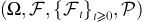satisfying the usual conditions, where Ω denotes a sample space,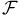denotes a σ-algebra,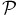denotes a probability measure and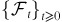denotes a filtration.

The risk-free stock price process can be described as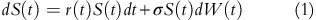where S(t) denotes the stock price, r(t) denotes the risk-free interest rate, σ denotes the volatility, W(t) denotes a Wiener process.

The expectation of Equation (1) is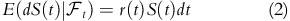where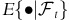is the expectation conditional on the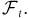Equation (2) tells us that in a risk-neutral world, the expected return on a risk-free stock is the risk-free interest rate r(t), that is, the discounted stock price under the risk neutral measure is a martingale process.

Next, we turn to a defaultable stock. The defaultable stock process proposed by Davis and Lischka (1999), Andersen and Buffum (2004) and Bloomberg (2009), and so on is given by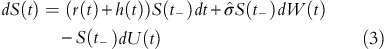where U(t) is an independent Poisson process with dU(t)=1 with probability h(t)dt and 0 otherwise, h(t) is the hazard rate or the default intensity, S(t_) is the stock price immediately before any jump at time t. The expectation of dU(t) is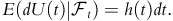The expectation of Equation (3) is given by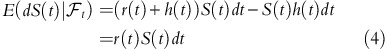It is shown in Equation (4) that the expected return of a defaultable stock under a jump-diffusion model also grows at the risk-free interest rate. Equation (3) is a simpler version of the Merton’s jump-diffusion model where the number of jumps is 1.

The jump-diffusion model was first proposed in the context of market risk, which naturally exhibits high skewness and leptokurtosis levels and captures the so-called implied volatility smile or skew effects. Ederington and Lee (1993) find that the markets tend to have overreaction and underreaction to the outside news. The jump part of the model can be interpreted as the market response to outside news. If there is not any outside news, the asset price changes according to a geometric Brownian motion. Since the market price of risk and risk preferences are irrelevant to asset pricing within the market risk context, the expected rate of return to the stockholders is equal to the risk-free rate under a risk-neutral measure.

However, we wonder whether it is appropriate to propagate the jump-diffusion model directly from the market risk domain to the credit risk domain, as credit risk actually impacts the valuation of assets. This is why financial institutions are required by regulators to report CVA. In fact, we will show in the following derivation that the expected return of a defaultable asset under a risk-neutral measure is actually equal to a risky rate instead of the risk-free rate. This conclusion is very important for risky valuation.

The world of credit modeling is divided into two main approaches: structural models and reduced-form (or intensity) models. The structural models regard default as an endogenous event, focusing on the capital structure of a firm. The reduced-form models do not explain the event of default endogenously, but instead characterize it exogenously as a jump process. In general, structural models are based on the information set available to the firm's management, such as the firm’s assets and liabilities, while reduced-form models are based on the information set available to the market, such as the firm’s bond prices or credit default swap (CDS) premia. Many practitioners in the credit trading arena have tended to gravitate toward the reduced-from models given their mathematical tractability. The reduced-form models can be made consistent with the risk-neutral probabilities of default backed out from corporate bond prices or CDS spreads/premia.

In the reduced-form models, the stopping (or default) time τ of a firm is modeled as a Cox arrival process (also known as a doubly stochastic Poisson process) whose first jump occurs at default and is defined as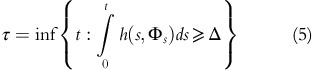where h(t) or h(t, Φt) denotes the stochastic hazard rate or arrival intensity dependent on an exogenous common state Φt, and Δ is a unit exponential random variable independent of Φt.

It is well-known that the survival probability from time t to s in this framework is defined by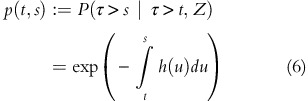The default probability for the period (t,s) in this framework is given by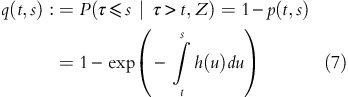We consider a defaultable asset that pays nothing between dates t and T. Let V(t) and V(T) denote its values at t and T, respectively. Risky valuation can be generally classified into two categories: the default time approach (DTA) and the default probability (intensity) approach (DPA).

The DTA involves the default time explicitly. If there has been no default before time T (that is, τ>T), the value of the asset at T is V(T). If a default happens before T (that is, t<τT), a recovery payoff is made at the default time τ as a fraction of the market valueFootnote 2 given by ϕV(τ) where ϕ is the default recovery rate and V(τ) is the market value at default. Under a risk-neutral measure, the value of this defaultable asset is the discounted expectation of all the payoffs and is given by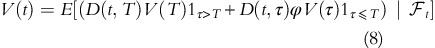where 1Y is an indicator function that is equal to 1 if Y is true and 0 otherwise, and D(t, τ) denotes the stochastic risk-free discount factor at t for the maturity τ given by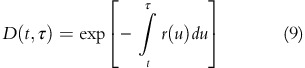Although the DTA is very intuitive, it has the disadvantage that it explicitly involves the default time/jump. We are very unlikely to have complete information about a firm’s default point, which is often inaccessible. Moreover, in a derivative transaction, the market-value-at-default V(τ) usually reflects the replacement cost of the transaction, where the replacement is also defaultable.Footnote 3 Therefore V(τ) should be determined via another risky valuation and so forth. Usually, valuation under the DTA is performed via Monte Carlo simulation.

The DPA relies on the probability distribution of the default time rather than the default time itself. We divide the time period (t, T) into n very small time intervals (Δt) and assume that a default may occur only at the end of each very small period. In our derivation, we use the approximation exp(y)≈1+y for very small y. The survival and default probabilities for the period (t, tt) are given by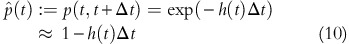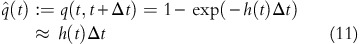The binomial default rule considers only two possible states: default or survival. For the one-period (t, tt) economy, at time tt the asset either defaults with the default probability q(t, tt) or survives with the survival probability p(t, tt). The survival payoff is equal to the market value V(tt) and the default payoff is a fraction of the market value: ϕ(tt)V(tt). Under a risk-neutral measure, the value of the asset at t is the expectation of all the payoffs discounted at the risk-free rate and is given by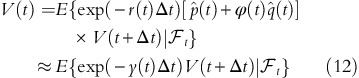where y(t)=r(t)+h(t)(1−ϕ(t))=r(t)+c(t) denotes the risky rate and c(t)=h(t)(1−ϕ(t)) is called the (short) credit spread.

Similarly, we have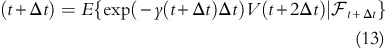Note that exp(−y(tt) is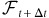-measurable. By definition, an-measurable random variable is a random variable whose value is known at time tt. On the basis of the taking out what is known and tower properties of conditional expectation, we have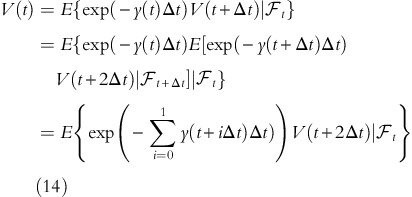By recursively deriving from t forward over T and taking the limit as Δt approaches 0, the risky value of the asset can be expressed as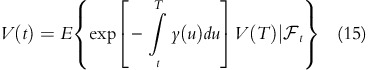Using the DPA, we obtain a closed-form solution for pricing an asset subject to credit risk. Another good example of the DPA is the CDS model proposed by J.P. Morgan (1999).

The derivation of Equation (15) takes into account all credit characteristics: possibility of a jump to default and recovery rate. It tells us that a defaultable asset under the risk-neutral measure grows at a risky rate. The risky rate is equal to a risk-free interest rate plus a credit spread. If the asset is a bond, the equation is the same as Equation (10) in Duffie and Singleton (1999), which is the market model for pricing risky bonds. The market bond model says that the value of a risky bond is obtained by discounting the promised payoff using the risk-free interest rate plus the credit spread.Footnote 4

Under a risk-neutral measure the market price of risk and risk preferences are irrelevant to asset pricing (Hull, 2003), and thereby the expectation of a risk-free asset grows at the risk-free interest rate. However, credit risk actually has a significant impact on asset prices. This is the reason that regulators, such as IASB and BCBS, require financial institutions to report a CVA in addition to the risk-free MTM value to reflect credit risk.

In asset pricing theory, the fundamental no-arbitrage theorems do not require expected returns to be equal to the risk free rate, but only that prices are martingales after discounting under the numeraire. For risk-free valuation, people commonly use a risk-free bond as the numeraire, whereas for risky valuation, they should choose an associated risky numeraire to reflect credit risk. The expected return is that of the numeraire.

If a company files bankruptcy, both bonds and stocks go into a default status. In other words, the default probabilities for both of them are the same (that is, equal to the firm’s probability of default). But the recovery rates are different because the stockholders are the lowest priority in the list of the stakeholders in the company, whereas the bondholders have a higher priority to receive a higher percentage of invested funds. The default proceedings provide a justification for our modeling assumptions: different classes of securities issued by the same company have the same default probability but different recovery rates.

According to Equation (15), we propose a risky model that embeds the probability of the default jump rather than the default jump itself into the price dynamics of an asset. The stochastic differential equation of a defaultable stock is defined as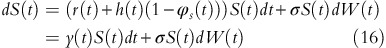where ϕs is the recovery rate of the stock and y(t)=r(t)+h(t)(1−ϕs(t)) is the risky rate.

For most practical problems, zero recovery at default (or jump to 0) is unrealistic. For example, the stock of Lehman Brothers fell 94.3 per cent on 15 September 2008 after the company filed for Chapter 11 bankruptcy. Similarly, the shares of General Motors plunged 32 per cent on 1 June 2009 after the firm initiated Chapter 11 bankruptcy. A good framework should flexibly allow people to incorporate different recovery assumptions into risky valuation.

Equation (16) is the direct derivation of Equation (15). The formula allows different assumptions concerning recovery on default. In particular, ϕs=0 represents the situation where the stock price jumps to 0, and ϕs=1 corresponds to the risk-free case. The expectation of Equation (16) is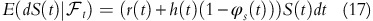Equation (17) says that the expected return of a stock subject to credit risk is equal to a risky rate rather than the risk-free rate. The risky rate reflects the compensation investors receive for bearing credit risk.

## PDE ALGORITHM

The numerical solution of our risky model can be obtained by either PDE methods, tree approaches or Monte Carlo simulation. In this article, we introduce the PDE procedure, but of course the methodology can be easily extended to the tree/lattice or Monte Carlo algorithms.

The defaultable stock price process is given by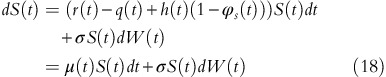where q(t) is the dividend and μ(t)=r(t)−q(t)+h(t)(1−ϕs(t))

The valuation of a convertible bond normally has a backward nature as there is no way of knowing whether the convertible should be converted without knowledge of the future value. Only on the maturity date, the value of the convertible and the decision strategy are clear. If the convertible is certain to be converted, it behaves like a stock. If the convertible is not converted at an intermediate node, we are usually uncertain whether the continuation value should be treated as a bond or a stock, because in backward induction the current value takes into account the results of all future decisions and some future values may be dominated by the stock or by the bond or by both. Therefore, we arrange the valuation so that the value of the convertible at each node is divided into two components: a component of bond and a component of stock, that is, L(S, t)=G(S, t)+B(S, t) where G(S, t) denotes the equity part of the convertible bond and B(S, t) denotes the bond part of the convertible.

Suppose that G(S, t) is some function of S and t. Applying Ito’s Lemma, we have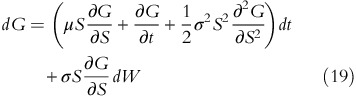Since the Wiener process underlying S and G are the same, we can construct the following portfolio so that the Wiener process can be eliminated.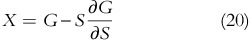Therefore, we have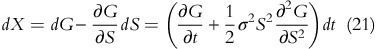In contrast to all previous studies, we believe that the defaultable equity should grow at the risky rate of the equity including dividends, whereas the equity part of the convertible bond should earn the risky rate of the equity excluding dividends, that is,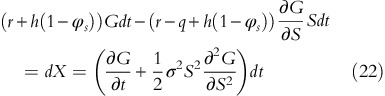So that the PDE of the equity component is given by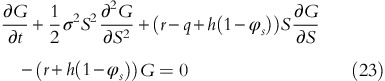Similarly, applying Ito’s Lemma to the bond part of the convertible B(S, t), we obtain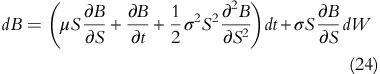Let us construct a portfolio so that we can eliminate the Wiener process as follows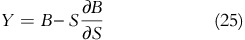Thus, we have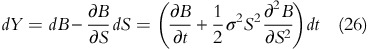The defaultable equity should grow at the risky rate of the equity including dividends, while the bond part of the convertible bond grows at the risky rate of the bond. Consequently, we have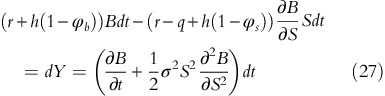where ϕb is the recovery rate of the bond.

The PDE of the bond component is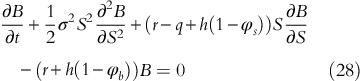Equations (23) and (28) are coupled through appropriate final and boundary conditions reflecting the terms and conditions of each individual convertible and need to be solved simultaneously. Convertible bonds often incorporate various additional features, such as call and put provisions.

The final conditions at maturity T can be generalized as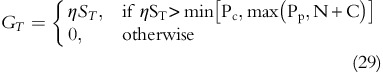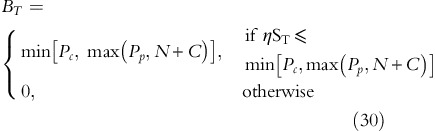where N denotes the bond principal, C denotes the coupon, Pc denotes the call price, Pp denotes the put price and η denotes the conversion ratio. The final conditions tell us that the convertible bond at the maturity is either a debt or an equity.

The upside constraints at time t∈[0, T] are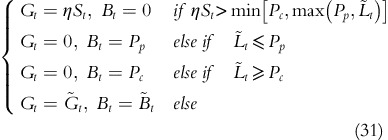where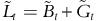is the continuation value of the convertible bond,is the continuation value of the bond component and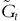is the continuation value of the equity component. Equation (31) says that the convertible is either in the continuation region or one of the three constraints (called put or converted). One can use finite difference methods to solve the PDEs (23) and (28) for the price of a convertible bond.

## EMPIRICAL RESULTS

This section presents the empirical results. We use 2 years of daily data from 10 September 2010 to 10 September 2012, that is, a total of 522 observation days. This proprietary data are obtained from an investment bank. They consist of convertible bond contracts, market observed convertible prices, interest rate curves, credit curves, stock prices, implied Black–Scholes volatilities and recovery rates.

We only consider the convertibles outstanding during the period and with sufficient pricing information. As a result, we obtain a final sample of 164 convertible bonds and a total of 164 × 522=85 608 observations. None of the convertibles in this sample actually defaulted during the time window.

As of 10 September 2012, the sample represents a family of convertible bonds with maturities ranging from 2 months to 36.6 years, and has an average remaining maturity of 4.35 years. The histogram of contracts on 10 September 2012 for various maturity classes is given in Figure 1.Figure 1

Convertible bond prices observed in the market will be compared with theoretical prices under different volatility assumptions. The sample is segmented into two sets according to maturities: a short-maturity class (0~8 years) and a long-maturity class (>8 years). We first select a convertible bond from each group: a 7-year (or 5-year outstanding) contract and a 20-year (or 17-year outstanding) contract shown in Table 1.

Let valuation date be 10 September 2012. An interest rate curve is the term structure of interest rates, derived from observed market instruments that represent the most liquid and dominant interest rate products for certain time horizons. Normally the curve is divided into three parts. The short end of the term structure is determined using the London Interbank Offered Rates (LIBOR). The middle part of the curve is constructed using Eurodollar futures that require convexity adjustments. The far end is derived using mid-swap rates. The LIBOR-future-swap curve is presented in Table 2. We bootstrap the curve and get the continuously compounded zero rates.

The equity information and recovery rates are provided in Table 3. To determine hazard rates, we need to know the observed market prices of corporate bonds or CDS premia, as the market standard practice is to fit the implied risk-neutral default intensities to these credit-sensitive instruments. The corporate bond prices are unfortunately not available for companies X and Y, but their CDS premia are observable as shown in Table 4. Usually the CDS market leads the bond market, in particular during crisis situation. Liquidity in the bond market is typically drying up during a financial crisis. Demand for insurance against default risk, on the other hand, increases if the issuer is experiencing financial stress. Consequently, prices and spreads derived from the CDS market tend to be more reliable. Said differently, CDSs on reference entities are often more actively traded than bonds issued by the same entities.

Unlike other studies that use bond spreads for pricing (see Tsiveriotis and Fernandes, 1998; Ammann et al, 2003; Zabolotnyuk et al, 2010; and so on), we perform risky valuation based on credit information extracted from CDS spreads. Given the recovery rates and the CDS premia, we can compute the hazard rates via a standard calibration process (J.P. Morgan, 2001).

The most important input parameter to be determined is the volatility for valuation. A common approach in the market is to use ATM-implied Black–Scholes volatilities to price convertible bonds. For the 5-year outstanding convertible bond (Case 1 in Table 1), we find the ATM implied Black–Scholes volatility is 31.87 per cent, and then price the convertible bond accordingly. The results are shown in Table 5. Our analysis actually indicates an overpricing of 0.42 per cent.

For the 17-year outstanding convertible bond (Case 2 in Table 1), however, most liquid stock options have relatively short maturates (rarely more than 8 years). Therefore, some authors, such as Ammann et al (2003), Loncarski et al (2009), Zabolotnyuk et al (2010), have to make do with historical volatilities. Similarly, we calculate the historical volatility as the annualized standard deviation of the daily log returns over the last 2 years (from 10 September 2010 to 10 September 2012), and then value the convertible bond based on this real-world volatility. The result shown in Table 5 reports an underpricing of 1.07 per cent. The test results demonstrate that the model prices are very close to the market prices.

We repeat this exercise for all contracts on all observation dates. For any short-maturity convertible bond, we use the ATM implied Black–Scholes volatility for pricing, whereas for any long-maturity convertible bond, we perform valuation via the historical volatility. The results are presented in Table 6.

Next, our sample is partitioned into subsamples according to the moneyness of convertibles. The moneyness is measured by the ratio of the conversion value to the equivalent straight bond value or the investment value. The underpricing of each daily observation with respect to the degree of moneyness is shown in Table 7, where moneyness between 0 and 0.9 corresponds to out-of-the-money; moneyness between 0.9 and 1.1 represents around-the-money; and moneyness higher than 1.1 is related to in-the-money.

From Table 7, it can be seen that the model prices fluctuate randomly around the market prices (sometimes overpriced and sometimes underpriced), indicating the model is quite accurate. Empirically, we do not find support for presence of a systematic underpricing as indicated in previous studies (see Carayannopoulos and Kalimipalli, 2003; Ammann et al, 2003; and so on). If there is no underpricing, how has the arbitrage strategy been successful in the past? Maybe convertible arbitrage is not solely based on underpricing.

In a typical convertible bond arbitrage strategy, the arbitrageur entails purchasing a convertible bond and selling the underlying stock to create a delta-neutral position. The number of shares sold short usually reflects a delta-neutral or market-neutral ratio. It is well known that delta-neutral hedging not only removes small directional risks but also is capable of making a good profit on an explosive upside or downside breakout if the position’s gamma is kept positive. As such, delta-neutral hedging is great for uncertain stocks that are expected to make huge breakouts in either direction. Since convertible bonds are issued mainly by start-up or small companies, the chance of a large movement in either direction is very likely. Even for very small movements in the underlying stock price, profits can still be generated from the yield of the convertible bond and the interest rebate for the short position.

We calculate the delta and gamma values for the two deals described in Table 1. The Greek versus spot equity price graphs are plotted in Figures 2, 3, 4, 5. It can be seen that the deltas increase with the underlying stock prices in Figures 2 and 4. At low market levels, the convertibles behave like their straight bonds with very small deltas. As the stock price increases, conversion becomes more likely. At certain market levels the convertibles are certain to be converted. In this case, the convertibles are similar to the underlying equities and the deltas are equal to the number of shares (that is, conversion ratios).Figure 2Figure 3Figure 4Figure 5

The gamma diagrams in Figures 3 and 5 have a frown shape. The gammas are the highest when the convertibles are at-the-money. It is intuitive that when the stock prices rise or fall, profits increase because of favorably changing deltas. For this reason, convertible bonds are very good candidates for delta-neutral hedging. Relatively large positive gammas of convertibles could be one of the main drivers of profitability in convertible arbitrage.

## CONCLUSION

This article aims to price hybrid financial instruments (for example, convertible bonds) whose values may simultaneously depend on different assets subject to credit risk in a proper and consistent way. The motivation for our model comes from the factor that if a company goes bankrupt, all the securities (including the equity) of the company default. The recovery is realized in accordance with the priority established by the Bankruptcy Code. In other words, different securities have the same probability of default, but different recovery rates.

Our study shows that risky asset pricing is quite different from risk-free asset pricing. In fact, the expectation of a defaultable asset actually grows at a risky rate rather than the risk-free rate. This conclusion is vital for risky valuation.

We propose a hybrid framework to value risky equities and debts in a unified way. The model relies on the probability distribution of the default jump rather than the default jump itself. As such, the model can achieve a high order of accuracy with a relatively easy implementation.

Empirically, we do not find evidence supporting a systematic underpricing hypothesis. We also find that convertible bonds have relatively large positive gammas, implying that convertible arbitrage can make a significant profit on a large upside or downside movement in the underlying stock price.

1. 1.

Here, risk-free means free of credit risk, but not necessarily of market risk

2. 2.

Here we use the recovery of market value assumption.

3. 3.

Many people in the market use the risk-free value as the market-value-at-default, which is inappropriate as any contract in the OTC market is risky when taking counterparty risk into account.

4. 4.

There is a liquidity component in the bond spread. This article, however, focuses on credit risk only.

## References

1. Agarwal, V., Fung, W., Loon, Y. and Naik, N. (2007) Liquidity provision in the convertible bond market: Analysis of convertible arbitrage hedge funds. CFR-Working Paper, Georgia State University.

2. Ammann, M., Kind, A. and Wilde, C. (2003) Are convertible bonds underpriced? An analysis of the French market. Journal of Banking & Finance 27 (4): 635–653.

3. Ammann, M, Kind, A. and Wilde, C. (2008) Simulation-based pricing of convertible bonds. Journal of Empirical Finance 15 (2): 310–331.

4. Andersen, L. and Buffum, D. (2004) Calibration and implementation of convertible bond models. Journal of Computational Finance 7 (4): 1–34.

5. Ayache, E., Forsyth, P.A. and Vetzal, K.R. (2003) The valuation of convertible bonds with credit risk. Journal of Derivatives 11 (1): 9–30.

6. Batta, G., Chacko, G. and Dharan, B. (2010) A liquidity-based explanation of convertible arbitrage alphas. Journal of Fixed Income 20 (1): 28–43.

7. Bloomberg. (2009) OVCV model description. Quantitative research and development, Equities team.

8. Brennan, M. and Schwartz, E. (1980) Analyzing convertible bonds. Journal of Financial and Quantitative Analysis 15 (4): 907–929.

9. Calamos, N.P. (2011) Convertible Arbitrage: Insights and Techniques for Successful Hedging. John Wiley & Sons.

10. Carr, P. and Linetsky, V. (2006) A jump to default extended CEV model: An application of Bessel processes. Finance and Stochastics 10 (3): 303–330.

11. Carayannopoulos, P. and Kalimipalli, M. (2003) Convertible bond prices and inherent biases. Journal of Fixed Income 13 (3): 64–73.

12. Choi, D., Getmansky, M. and Tookes, H. (2009) Convertible bond arbitrage, liquidity externalities, and stock prices. Journal of Financial Economics 91 (2): 227–251.

13. Davis, M. and Lischka, F.R. (1999) Convertible bonds with market risk and credit risk. Working paper, Tokyo, Japan, Mitsubishi International plc.

14. Duffie, D. and Huang, M. (1996) Swap rates and credit quality. Journal of Finance 51 (3): 921–949.

15. Duffie, D. and Singleton, K.J. (1999) Modeling term structure of defaultable bonds. Review of Financial Studies 12 (4): 687–720.

16. Ederington, L. and Lee, H. (1993) How markets process information: News releases and volatility. Journal of Finance 48 (4): 1161–1191.

17. Grimwood, R. and Hodges, S. (2002) The valuation of convertible bonds: A study of alternative pricing models. Working paper, Warwick University.

18. Goldman Sachs. (1994) Valuing convertible bonds as derivatives. Quantitative Strategies Research Notes, Goldman Sachs.

19. Hull, J. (2003) Options, Futures and Other Derivatives, 5th edn. Upper Saddle River, NJ: Prentice Hall.

20. Jarrow, R.A. and Protter, P. (2004) Structural versus reduced form models: A new information based perspective. Journal of Investment Management 2 (2): 34–43.

21. Loncarski, I., Horst, J. and Veld, C. (2009) The rise and demise of the convertible arbitrage strategy. Financial Analysts Journal 65 (5): 35–50.

22. Merton, R.C. (1976) Option pricing when underlying stock returns are discontinuous. Journal of Financial Economy 3 (1–2): 125–144.

23. J.P. Morgan. (1999) The J. P. Morgan Guide to Credit Derivatives. Risk Publications.

24. J.P. Morgan. (2001) Par Credit Default Swap Spread Approximation from Default Probabilities. Risk Publications.

25. Somanath, V.S. (2011) International Financial Management. I.K. International Publishing House.

26. Tsiveriotis, K. and Fernandes, C. (1998) Valuing convertible bonds with credit risk. Journal of Fixed Income 8 (4): 95–102.

27. Xiao, T. (2013) An accurate solution for credit value adjustment (CVA) and wrong way risk. Working Paper, BMO.

28. Zabolotnyuk, Y., Jones, R. and Veld, C. (2010) An empirical comparison of convertible bond valuation models. Financial Management 39 (2): 675–705.

Download references

## Author information

Authors

### Corresponding author

Correspondence to Tim Xiao.

## APPENDIX

### APPENDIX

In this section, we describe the numerical method used to solve discrete forms of (23) and (28). Let x=ln(St/S0) and define backward time as δ=Tt. Equations (23) and (28) can be rewritten as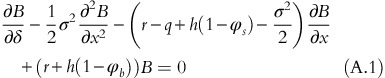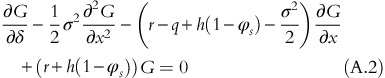Equations (A.1) and (A.2) can be approximated using Crank–Nicolson rule. We discretize the x to be equally spaced as a grid of M nodes. At the maturity, GT and BT are determined according to (29) and (30). At any time i+1, the boundary conditions are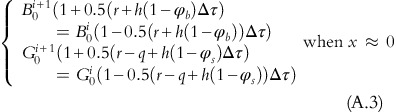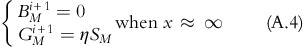Then, we conduct the backward induction. The procedure is as follows.

For i=penultimateTime to currentTime

// determine accrual interest and call/put prices

// determine boundary nodes

// use the PSOR (Projected Successive over Relaxation) method to obtain the continuation value of the bond component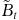and the continuation value of the equity component, applying the constraint (31).

The value at node[y] is the convertible bond price where the equity price at node[y] is equal to the current market stock price.

## Rights and permissions

Reprints and Permissions

## About this article

### Cite this article

Xiao, T. A simple and precise method for pricing convertible bond with credit risk. J Deriv Hedge Funds 19, 259–277 (2013). https://doi.org/10.1057/jdhf.2014.5

Download citation

• Received:

• Revised:

• Published:

• Issue Date:

### Keywords

• hybrid financial instrument
• convertible bond
• convertible underpricing
• convertible arbitrage
• default time approach (DTA)
• default probability approach (DPA)
• jump diffusion